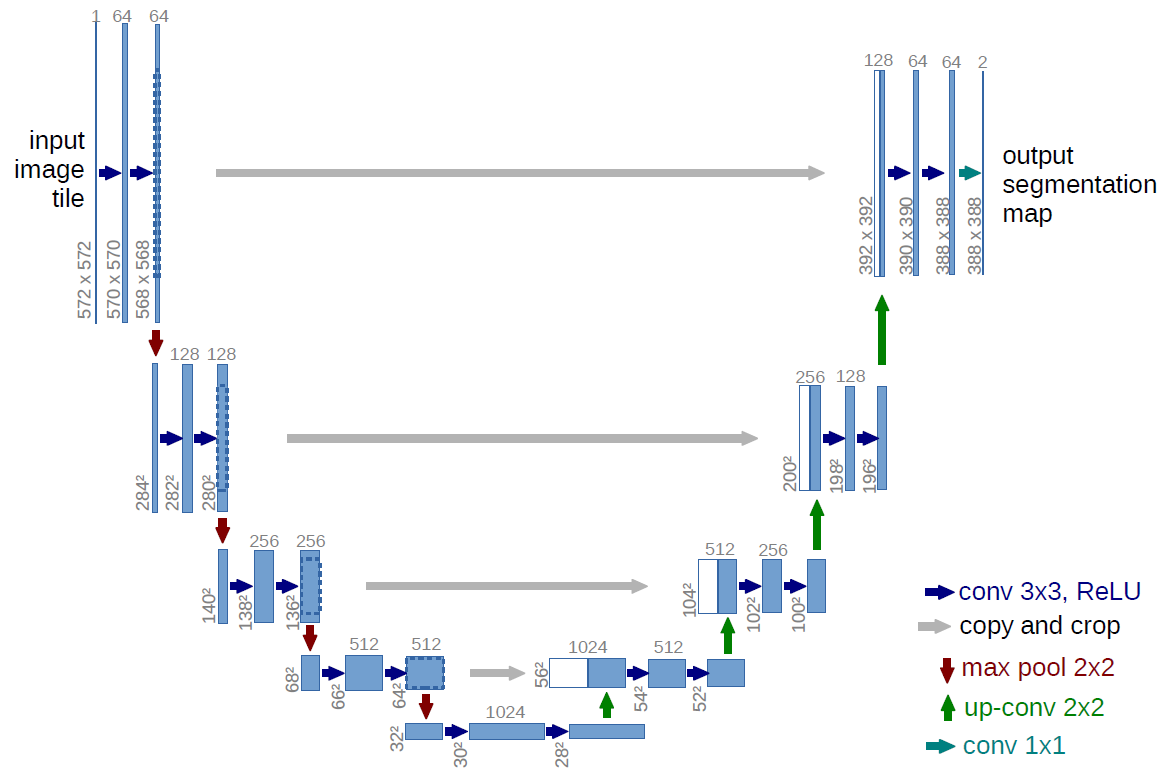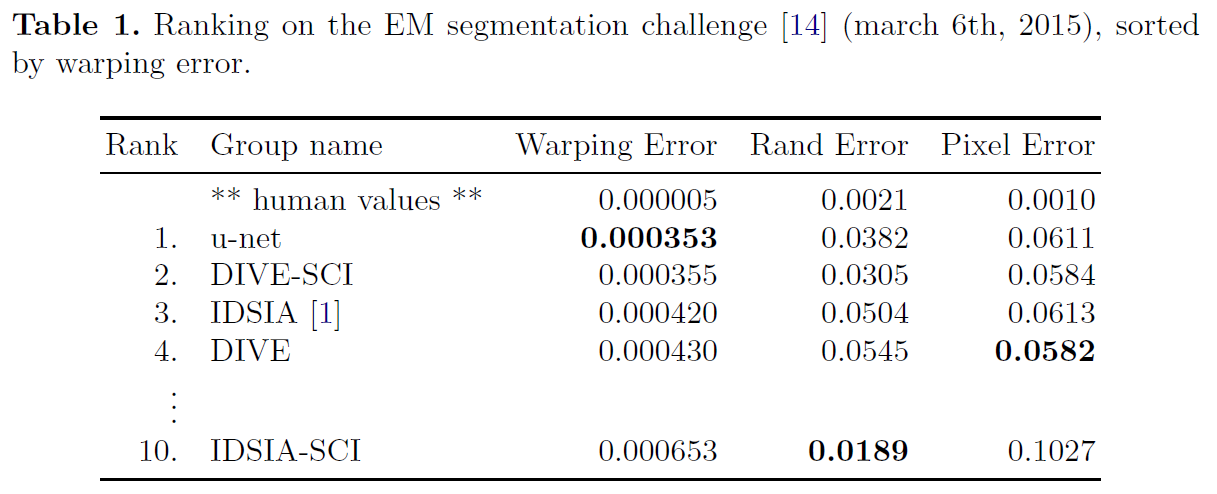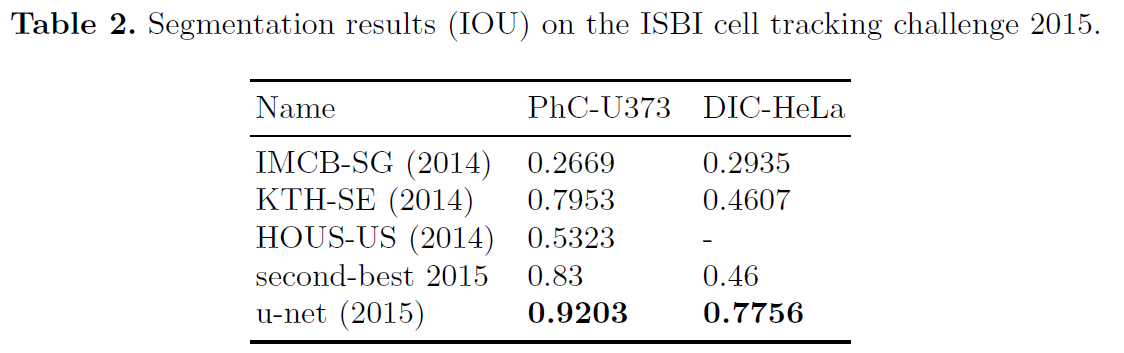“U-Net: Convolutional Networks for Biomedical Image Segmentation” is a famous segmentation model not only for biomedical tasks and also for general segmentation tasks, such as text, house, ship segmentation.

# Summary

• Proposed Solution
• Present a network and training strategy that relies on the strong use of data augmentation to use the available annotated samples more efficiently.
• The architecture consists of a contracting path to capture context and a symmetric expanding path that enables precise localization.
• Contribution
• U-net can be trained end-to-end from very few images and outperforms the prior best method on the ISBI challenge for segmentation of neuronal structures in electron microscopic stacks.
• It is fast, segmentation of a 512x512 image takes less than a second on a recent GPU.

# U-NetFigure 1: U-net architecture(example for 32x32 pixels in the lowest resolution). Each blue box corresponds to a multi-channel feature map. The number of channels is denoted on top of the box. The x-y-size is provided at the lower left edge of the box. White boxes represent copied feature maps. The arrows denote the different operations.

## Network Architecture

U-net consits of a contracting path (left side) and an expansive path (right side).

• Contracting path
• typical architecture of a convolutional network
• repeated application of two 3x3 convolutions (unpadded convolutions)
• each followed by a rectified linear unit (ReLU) and a 2x2 max pooling operation with stride 2 for downsampling
• at each downsampling step, we double the number of feature channels
• Expansive path
• consists of an upsampling of the feature map followed by a 2x2 convolution (“up-convolution”) that halves the number of feature channels
• a concatenation with the correspondingly cropped feature map from the contracting path, and two 3x3 convolutions
• each followed by a ReLU
• the cropping is necessary due to the loss of border pixels in every convolution

At the final layer a 1x1 convolution is used to map each 64-component feature vector to the desired number of classes. In total the network has 23 convolutional layers.

## Training

The energy function is computed by a pixel-wise soft-max over the final feature map combined with the cross entropy loss function. The soft-max is defined as

The cross entropy then penalizes at each position the deviation of $p_{l(x)}(x)$ from 1 using

The seperation border is computed using morphological operations. The weight map is then computed as

# Experiments